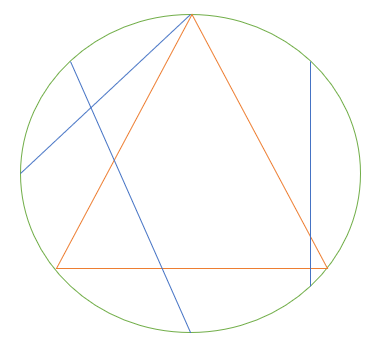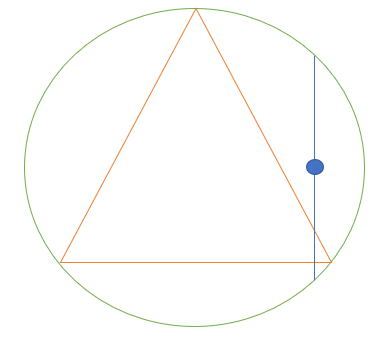# Bertrand Paradox: Definition & Example

Share on

Key Takeaways:

• The Bertrand Paradox, which concerns probabilities, isn’t actually a paradox at all.
• The problem is with wording, not with actual situations.
• An example can be illustrated with the “equilateral triangle drawn within a circle” problem.

## What is the Bertrand Paradox?

The Bertrand Paradox shows that if you don’t define probabilities well, then the mechanism that generates random variables will also not be well defined . This leads to a paradox—a confusing, contradictory situation.

Bertrand’s paradox was one of the reasons classical probability fell out of favor at the turn of the 20th century. However, it really isn’t a paradox at all; if you’re careful to define your probability spaces, then the paradox doesn’t exist.

In his 1889 treatise Calculs des Probabilities, Bertrand asked:

“If we choose at random two points on the surface of a sphere, what is the probability that the distance between them is less than 10?”

Bertrand offered two solutions.

1. The probability a certain segment of the sphere’s surface, containing the two points, is proportional to the segment’s area as a whole.
2. A given arc on a great circle , with the two points, is proportional to the length of the arc.

In fact, there are several ways to approach the problem. This leads to multiple solutions and probabilities.

To illustrate the paradox from a slightly different angle (pun intended), let’s take a look at an equilateral triangle drawn within a circle.An equilateral triangle (orange) inscribed in a circle. Three chords are shown in blue.

What we want to know is, if we pick a random chord (a line segment drawn between two points on the circumference), what are the odds the chord is longer than one side of the triangle?

The solution seems simple enough. Pick a random point in the circle, use that as a midpoint for a chord:This way of choosing a random chord gives a probability of 0.25 . But this obviously isn’t the only way to find a chord. We could choose a point on the perimeter to start with (that will give a probability of 2/3) or pick a random radius, then a random point (probability = ½).

All solutions are valid. However, they can’t all be valid at the same time—hence the paradox. The reason for the paradox is that the initial problem is ill-posed. We weren’t given any rules for choosing our chords, so that left the door open for multiple interpretations.

## References

 Chang, M. Paradoxes in Scientific Inference. CRC Press, 2012.
 Bartlett, P. (2014). Lecture 2: Bertrand’s Paradox. Retrieved June 2, 2020 from: http://web.math.ucsb.edu/~padraic/ucsb_2013_14/math7h_s2014/math7h_s2014_lecture2.pdf

CITE THIS AS:
Stephanie Glen. "Bertrand Paradox: Definition & Example" From StatisticsHowTo.com: Elementary Statistics for the rest of us! https://www.statisticshowto.com/bertrand-paradox/
---------------------------------------------------------------------------Need help with a homework or test question? With Chegg Study, you can get step-by-step solutions to your questions from an expert in the field. Your first 30 minutes with a Chegg tutor is free!